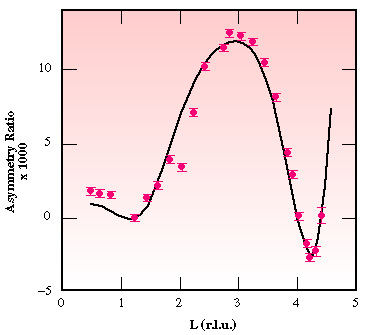Fig. 33: Asymmetry ratio R as a function of the reciprocal space co-ordinate L (which is proportional to the perpendicular momentum transfer) measured along the (H,K) = (1,0) direction, for a film of six atomic layers of Co deposited on the Pt substrate. The zero values of R at L = 1 and 4, where Bragg conditions are fulfilled, is an indication of a non-magnetised Pt atom in the bulk of the crystal. The non-zero values for 1 < L < 4 are due to a single atomic plane of magnetised Pt atoms: the one in contact with the cobalt film.

Back